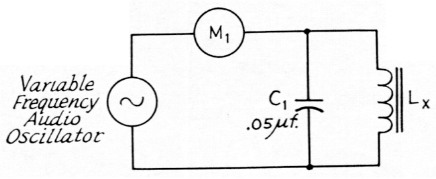# Finding the value of an unkwown inductance with an audio oscillator

Home - Techniek - Electronica - Radiotechniek - Radio amateur bladen - QST - Finding the value of an unkwown inductance with an audio oscillator

It's no chore to determine the value of an unknown inductance below 0.5 henry with a grid-dip meter and a known capacitance. Higher values of inductance require the use of a known capacitor so small in value as to make this method of measurement highly inaccurate. The circuit shown in Fig. 1 illustrates one method of measuring inductors of 0.1 henry to 100 henrys with good accuracy. A v.t.v.m. and an audio oscillator are used in place of the grid-dip meter. The v.t.v.m. is switched to a low a.c. scale, the oscillator adjusted for full-scale deflection of the voltmeter and the oscillator frequency varied for a dip in this reading. After noting the frequency at which the dip occurs, the value of the unknown inductance may be found by solving the formula listed below.Fig. 1. Circuit for determining the value of an unknown inductance.

 Lx Unknown inductance. M1 V.t.v.m., low a.c. scale.

Lower and higher values of inductance can also be measured by this method, but C1 will have to be changed accordingly to produce a resonant frequency within the range of the audio oscillator.

where
L = Unknown inductance in henrys.
π = 3.14.
C = Capacitance of C1 in farads.
Fr = Frequency of dip in cycles.

Noel B. Sargent, K8QQQ

(If the inductor is to be used in an application where no d.c. passes through the inductor, as in an audio filter, this method of measurement will be satisfactory. Another technique is necessary if the circuit requires direct current to flow through the inductance. See Ellison, "Measuring Inductance of D.C. Loaded Chokes," QST, February, 1963. - Editor.)